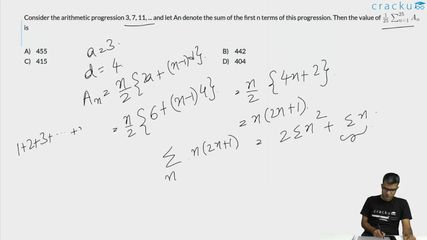Question 62

# Consider the arithmetic progression 3, 7, 11, ... and let $$A_n$$ denote the sum of the first n terms of this progression. Then the value of $$\frac{1}{25} \sum_{n=1}^{25} A_{n}$$ is

Solution

Sum of n terms in an A.P = $$\frac{n}{2}\left(2a+\left(n-1\right)d\right)$$

$$A_n=\frac{n}{2}\left(6+\left(n-1\right)4\right)=n\left(2n+1\right)$$

$$\Sigma\ A_n=\Sigma\ n\left(2n+1\right)=2\Sigma\ n^2+\Sigma\ n=\ \frac{\ 2n\left(n+1\right)\left(2n+1\right)}{6}+\ \frac{\ n\left(n+1\right)}{2}$$

Substituting n = 25, we get

$$\frac{1}{25} \sum_{n=1}^{25} A_{n}$$ = $$\frac{1}{25}\left(\ \frac{\ 2\left(25\right)\left(25+1\right)\left(50+1\right)}{6}+\ \frac{\ 25\left(25+1\right)}{2}\right)$$

$$\frac{1}{25} \sum_{n=1}^{25} A_{n}$$ = $$\ 26\left(17\right)+13$$ = 455

### View Video Solution